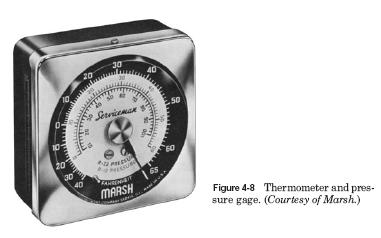# Temperature and Heat

The production of excess heat in a system will cause problems. Normally, matter expands when heated. This is the principle of thermal expansion. The linear dimensions increase, as does the volume. Removing heat from a substance causes it to contract in linear dimensions and in volume. This is the principle of the liquid in a glass thermometer.

Temperature is the measure of hotness or coldness on a definite scale. Every substance has temperature.

Molecules are always in motion. They move faster with a temperature increase and more slowly with a temperature decrease. In theory, molecules stop moving at the lowest temperature possible. This temperature is called absolute zero. It is approximately ?460°F (?273°C).

The amount of heat in a substance is directly related to the amount of molecular motion. The absence of heat would occur only at absolute zero. Above that temperature, there is molecular motion. The amount of molecular motion corresponds to the amount of heat.

The addition of heat causes a temperature increase. The removal of heat causes a temperature decrease. This is true except when matter is going through a change of state.

Heat is often confused with temperature. Temperature is the measurement of heat intensity. It is not a direct measure of heat content. Heat content is not dependent on temperature. Heat content depends on the type of material, the volume of the material, and the amount of heat that has been put into or taken from the material. For example, one cup of coffee at 200ºF (93.3°C) contains less heat than 1 gal of coffee at 200ºF (93.3°C). The cup at 200ºF (93.3°C) can also contain less heat than the gallon at a lower temperature of 180ºF (82.2°C).